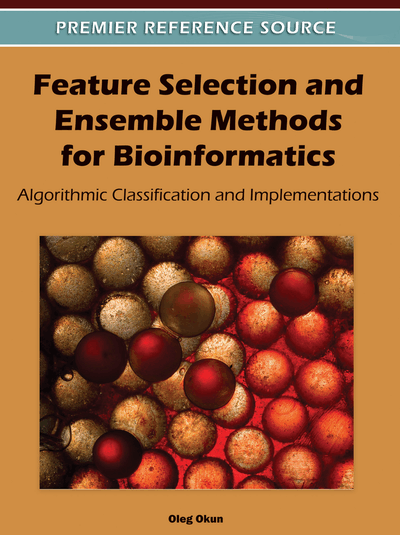# Extreme Value Distribution Based Gene Selection

DOI: 10.4018/978-1-60960-557-5.ch011
Available
\$37.50
No Current Special Offers

Top

## Blend Of Elements Of Extreme Value Theory And Logistic Regression

(Li, Sun, & Grosse, 2004) introduced the idea of using extreme value distribution theory for gene selection based on logistic regression. Each gene is modeled by means of logistic regression separately from other genes. This discriminant method is preferred over such a simple method as the t-test because logistic regression does not assume that gene expression levels are normally distributed. As a result, logistic regression is more robust to outliers than the t-test.

In statistical modeling, one of the central notions is the likelihood which is the probability of a set of observations given some parameter or parameters(Everitt, 2006). For a random sample consisting of n observations,with probability distribution, the likelihood is defined as (Everitt, 2006)

The maximum likelihood principle says that out of all possible values ofone should choose the one maximizing the likelihood L. This means that one needs first to write down the mathematical expression for L, then to take the derivativeand set the result to zero. Good examples of how to utilize the maximum likelihood principle in practice are provided in Chapter 2 in (Roff, 2006).

In statistical models such as logistic regression, the typical way to perform gene selection is to compare the maximum likelihood of the model given the real data and the expected maximum likelihood of the model given an ensemble of surrogate data with randomly permuted labels1. The computational bottleneck is the second likelihood due to the very large number of possible permutations. Li et al. proposed to replace this step with another one involving extreme value statistics based on which two gene selection criteria are introduced. In other words, in the approach of Li et al. the maximum likelihood of each gene in the real data is compared with the maximum likelihood of the top-ranking (hence, the extreme value theory emerges here) gene in the label-permuted data. Therefore, numerous calculations of single-gene likelihoods in the surrogate data are replaced with the calculation of the top-ranking gene likelihood carried out only once.

As follows from its name, this gene selection method rests on three components requiring definition: extreme values, extreme value distribution, and logistic regression.

## Complete Chapter List

Search this Book:
Reset